International
Tables for
Crystallography
Volume A
Space-group symmetry
Edited by Th. Hahn

International Tables for Crystallography (2006). Vol. A, ch. 8.1, pp. 723-724

## Section 8.1.5. Crystallographic symmetry operations

H. Wondratscheka*

aInstitut für Kristallographie, Universität, D-76128 Karlsruhe, Germany
Correspondence e-mail: hans.wondratschek@physik.uni-karlsruhe.de

### 8.1.5. Crystallographic symmetry operations

| top | pdf |

Crystallographic symmetry operations are special motions.

Definition: A motion is called a crystallographic symmetry operation if a crystal pattern exists for which it is a symmetry operation.

We consider a crystal pattern with its vector lattice L referred to a primitive basis. Then, by definition, each vector of L has integral coefficients. The linear part of a symmetry operation maps L onto itself: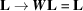. Since the coefficients of all vectors of L are integers, the matrix W is an integral matrix, i.e. its coefficients are integers. Thus, the trace of W,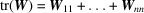, is also an integer. In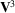, by reference to an appropriate orthonormal (not necessarily crystallographic) basis, one obtains another condition for the trace,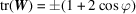, where ϕ is the angle of rotation or rotoinversion. From these two conditions, it follows that ϕ can only be 0, 60, 90, 120, 180° etc., and hence the familiar restriction to one-, two-, three-, four- and sixfold rotations and rotoinversions results.2 These results imply for dimensions 2 and 3 that the matrix W satisfies the condition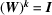, with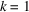, 2, 3, 4 or 6.3 Consequently, for the operation (W, w) in point space the relation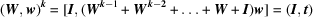holds.

For the motion described by (W, w), this implies that a k-fold application results in a translation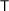(with translation vector t) of the crystal pattern. The (fractional) translation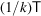is called the intrinsic translation part (screw or glide part) of the symmetry operation. Whereas the translation part' of a motion depends on the choice of the origin, the intrinsic translation part' of a motion is uniquely determined. The intrinsic translation vector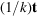is the shortest translation vector of the motion for any choice of the origin.

If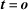, the symmetry operation has at least one fixed point and is a rotation, inversion, reflection or rotoinversion. If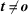, the termis called the glide vector (for a reflection) or the screw vector (for a rotation) of the symmetry operation. Both types of operations, glide reflections and screw rotations, have no fixed point.

For the geometric visualization of symmetry, the concept of symmetry elements is useful.4 The symmetry element of a symmetry operation is the set of its fixed points, together with a characterization of the motion. For symmetry operations without fixed points (screw rotations or glide reflections), the fixed points of the corresponding rotations or reflections, described by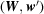with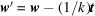, are taken. Thus, in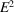, symmetry elements are N-fold rotation points (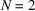, 3, 4 or 6), mirror lines and glide lines. In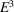, symmetry elements are rotation axes, screw axes, inversion centres, mirror planes and glide planes. A peculiar situation exists for rotoinversions (exceptand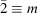). The symmetry element of such a rotoinversion consists of two components, a point and an axis. The point is the inversion point of the rotoinversion, and the axis of the rotoinversion is that of the corresponding rotation.

The determination of both the nature of a symmetry operation and the location of its symmetry element from the coordinate triplets, listed under Positions in the space-group tables, is described in Section 11.2.1 of Chapter 11.2.